This blog is part 2 of a 3 part series of implementing a gradient filter on an FPGA.  If you have not already read part2 see the link below to get up to speed before reading this blog.  Additionally the user can catch some of our previous blog posts, linked below.

Part1 of this blog series

Other FPGA blogs by ValentF(x)

## Introduction

Gradient filters for image processing are kernel based operations that process an array of pixels from an input image to generate a single pixel in an output image. Most gradient filter algorithms use a 3x3 pixel window but some uses a 2x2 pixel window. In this posts we will work on a Sobel based operator to implement the gradient filter. The Sobel kernel is designed to extract the gradient in an image, either over the U axis (along columns) or V axis (along horizontal lines) and the two U/V oriented filters can be combined to extract the gradient direction or the U/V gradient intensity.

Figure 1 : beagleboard.org logo and its gradient intensity, extracted using U/V Sobel filters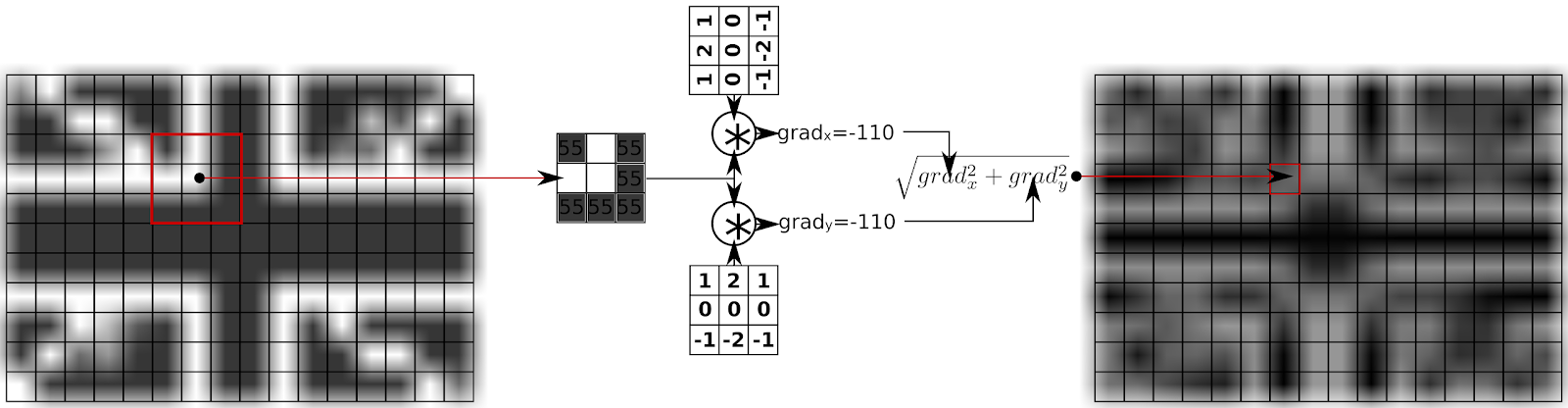Figure 2 : Computing gradient intensity for a small image

Gradient filter is a step for many vision processing tasks like  : edge enhancement, corner extraction, edge detection ...

## Problem analysis

In this part of the article we will analyze the Sobel filter for the V direction (rows) and then generalize to the U direction (columns).

The Sobel filter is a 2D spatial high-pass FIR filter (Sobel operator - Wikipedia, the free encyclopedia).  FIR Filter refers to Finite Impulse Response.  This class of filter computes the filter output based-on the input history, as opposed to an Infinite Impulse Response Filter (IIR) that computes the output based on input history and output history.

The filter kernel is composed of  the following: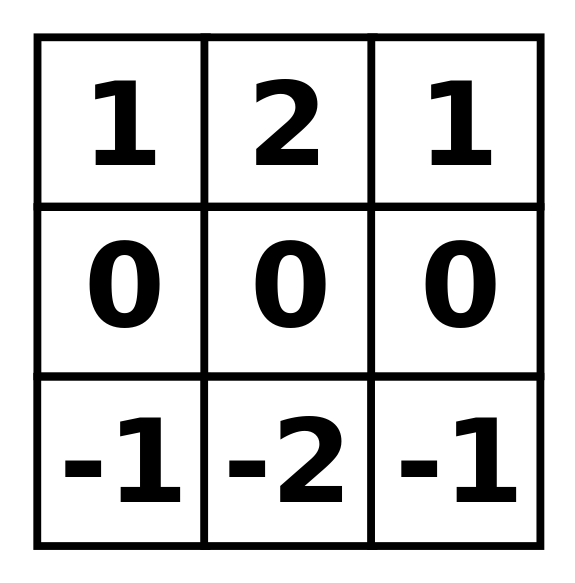This means that to generate a single pixel in the output image, we need to access 9 pixels in the input image, multiply them by 9 values and add the partial results. If Some kernel components are 0, only 6 multiplication and 5 addition operations are needed to be performed. This operation of multiplying the kernel values with values from the input to generate a single output, called a convolution and is the foundation of a wide range of kernel based operations.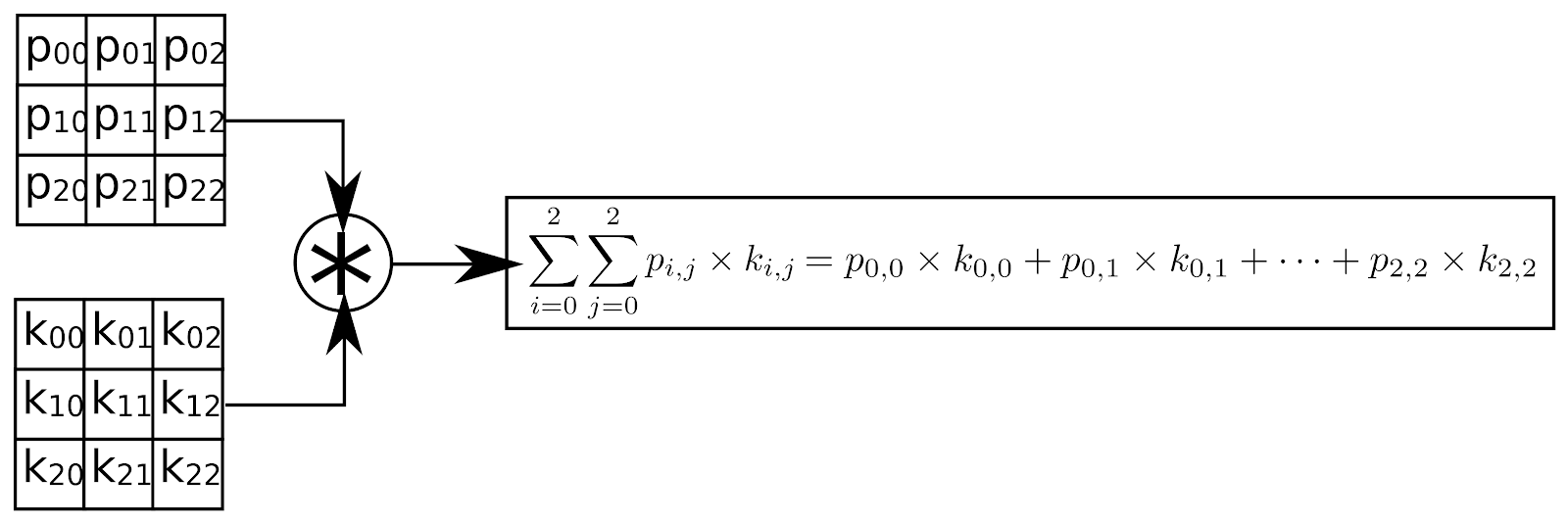Figure 2 : Convolution operation for a 3x3 kernel

To sum-up, the convolution operations requires :

• To be able to generate a 3x3 pixel window from the input image for every U,V positions in this image
• To perform 9 multiplications and 8 additions for every pixel (can be optimized depending on the kernel)

In a typical software implementation, the program has direct access to the complete image, which makes accessing the data a memory addressing problem to generate the 3x3 window. Based on our previous post, Gradient Filter implementation on an FPGA - Part 1 Interfacing an FPGA with a camera we only have access to a single pixel of the image at a given time so the window problem will require to manage a memory that stores a number of pixels of the pixel stream.

Hardware implementation

To develop our hardware Sobel filter, we will divide the architecture into two main modules, a 3x3 block module and the arithmetic module.

### 3x3 Block

As seen in the previous post, the camera module does not output a 2D stream of pixels, but rather a 1D stream of pixels with an additional signal indicating when to increment the second dimension (hsync). To design this component we first need to understand what is the smallest amount of data we need to store in order to do the filtering. Designing in an FPGA is, most of the time, a matter of designing the optimal architecture so every memory bit counts. A quick study of the problem shows that the minimum amount of data to store is two lines of the image plus 3 pixels to have a sliding 3x3 window in the image.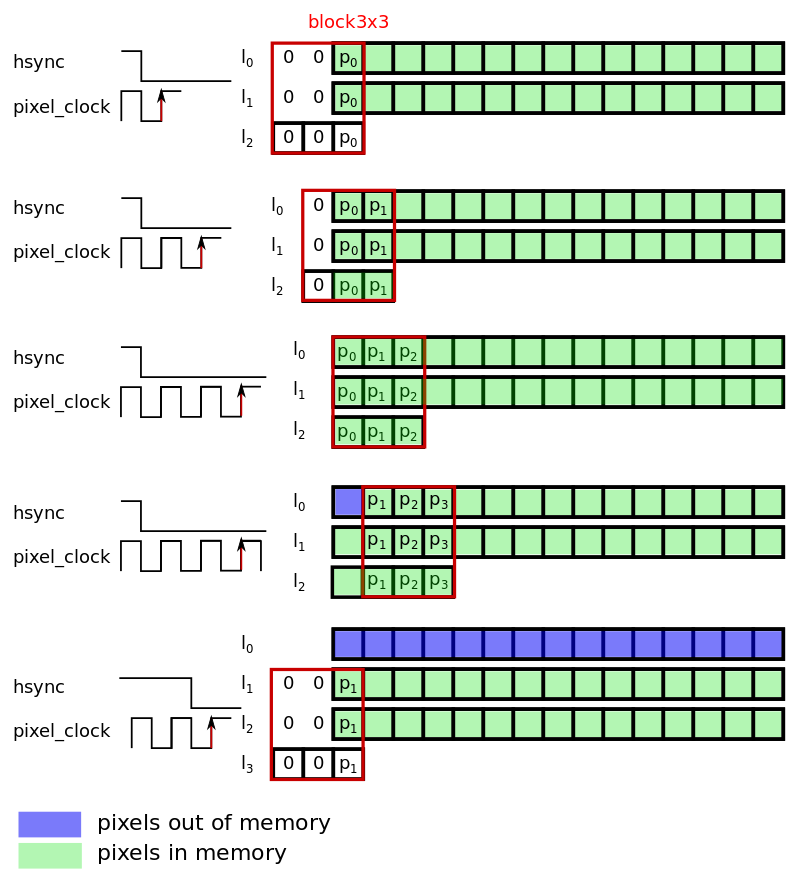Figure 3 : Position of the sliding windows based on the hsync and pixel_clock signals.

This memory management architecture can simply be performed in hardware. The 3x3 Block works on a 2D register of 3x3 size in each position containing a pixel (8-bit or 9-bit for signed pixels).  The steps for this procedure as are follows.

• Store pixel at index 2, 3 and index 3, 3 respectively in memory 1 and memory 2
• Shift columns of the block register
• Take the incoming pixel, store it in at index 3, 3
• Grab one pixel in memory 2  and store it a index 2, 3
• Grab one pixel in memory 1 and store it a index 1, 3

The address management of memory location 1 and 2 is performed based on the pixel count and line count extracted from the synchronization signals pixel_clock and hsync. The sequence of operations described previously can all be executed in parallel (screams to use an FPGA).  This first version of the 3x3 block requires very little work, and works fine at the pixel_clock frequency, but consumes too many memory units. Most of the time, block RAM (BRAM) in an FPGA are atomic so the synthesizer cannot map multiple memories described in HDL to a single block RAM in the FPGA (let say you describe a 256 Byte memory and a 64 Byte memory, they cannot be mapped to a single 2kByte memory).

Info:  In the Spartan3/6, block RAMs are 18Kbits with a configurable bus-width, these block RAMs can be split into two 9Kbits on the Spartan-6.

In our case, memory1 and memory2 cannot be mapped to the same block RAM and will use two block RAMs with lot of their spaces being unused (one line in VGA is 640 pixels and a block RAM can contain 1K pixels or 2K pixels). Where the addressing of the two memories being very similar (exactly similar in fact), one trick is to use a 16-bit wide memory and store pixels of memory1 in the MSBs of the data and pixels of memory2 in the LSBs of the data.

For the actual code, have at look at https://github.com/jpiat/hard-cv/blob/master/hw/rtl/image/block3X3.vhd

### Sobel arithmetic

Once we have the block of pixels for every input pixel, the pixel window needs to be convoluted with the Sobel kernel. Most elements of the kernel being zeros, this multiplication requires 6 multiplications. Small FPGAs have very few multipliers and all arithmetic optimization will greatly help. In the case of the Sobel filter, the optimization comes from the fact that all multiplications are ones and twos, so only shifting bits are required!  For a general implementation of the convolution, the convolution would require at least one multiplier (DSP block in the FPGA) and potentially up to 9 multipliers.   Considering that a Spartan-6 LX9 FPGA is composed of 16 DSP blocks, a non optimized implementation of a convolution filter can easily consume all DSP blocks of the FPGA.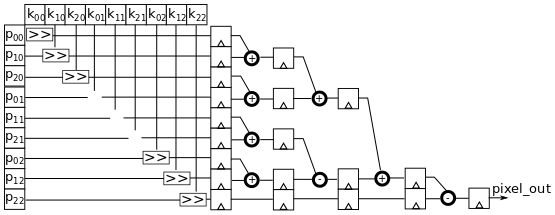Figure 4 : Optimized Sobel convolution computation with pipelined adder-tree

This kind of implementation is very frequency efficient and allows to target very high clock frequencies in the system. The main drawback is that it uses more registers, hence more area of the FPGA.

Now that we have the architecture we also need to configure the width of the data-path. One possible way for doing this is to perform all operations on 32-bits or 16-bits to avoid overflow. The better solution is to compute the actual length of the data along it’s path and only use the useful bits and hence limit the resource use of the system.

To Sum it all up - Steps Required:

• Input is a 8-bit pixels
• Pixels are multiplied by positive and negative values so needs to be coded on 9-bits at the arithmetic module input (signed binary arithmetic)
• Pixels are multiplied by 1, 2, 1, -1, -2, -1 so  are respectively shifted by 0, 1, 0, 0, 1, 0, which gives us for each partial product 9-bit, 10-bit, 9-bit, 9-bit, 10-bit, 9-bit
• These values are added - each addition can add up to 1-bit to the wider word : 9-bit + 10-bit = 11-bit, 9-bit+9-bit=10-bit, 9-bit + 10-bit = 11-bit => 11-bit + 10-bit + 11-bit = 13-bit

In fact because each addition cannot be considered separately, we can consider that the final addition of 11-bit + 10-bit + 11-bit will not overflow from 11-bits. Lets do the worst case to check that it works.

Worst case for a Sobel filter along the image lines is the following 3x3 pixels window (pixel in this case are unsigned values) :
255, 255, 255xxx,  xxx,  xxx000, 000, 000
1x255+2x255+1x255-1x0-2x0-1x0 = 1020,  fits on 10-bits + a bit for the sign => 11bits.

So the output of one Sobel convolution for the Sobel filter fits on 11-bits. To get the gradient intensity the U and V gradient needs to be combined using the Euclidean distance.  The Euclidean distance requires to perform the square of each gradient and then perform a square-root of the addition.
Square roots are very expensive to perform on an FPGA, so we can choose to approximate the Euclidean distance by summing the absolute values.  This saves quite a lot of FPGA area and improves the performance of the system.

There are two implementations

• First implementation uses a processing clock that is 4x the max pixel clock. In this implementation, the FPGA can use up to two clock cycles to process a single pixel. This is not particularly useful for Sobel, but some other convolutions may use a different kernel coefficient that requires multiplication instead of shifting. This implementation is fine for “small” resolutions like VGA at up to 60FPS (24MHz pixel-clock, 100MHz processing clock).
• Second implementation is fully pipelined and uses the pixel clock as the processing clock. This implementation uses more resources but is very efficient frequency wise (pixel-clock can be higher than 130MHz) and process HD images.

The next step will be the debugging of our filter using a specific test-bench to stimulate the design with real images and generate output images.  Stay tuned for part 3 of this blog series for implementing a test-bench and simulating the design.